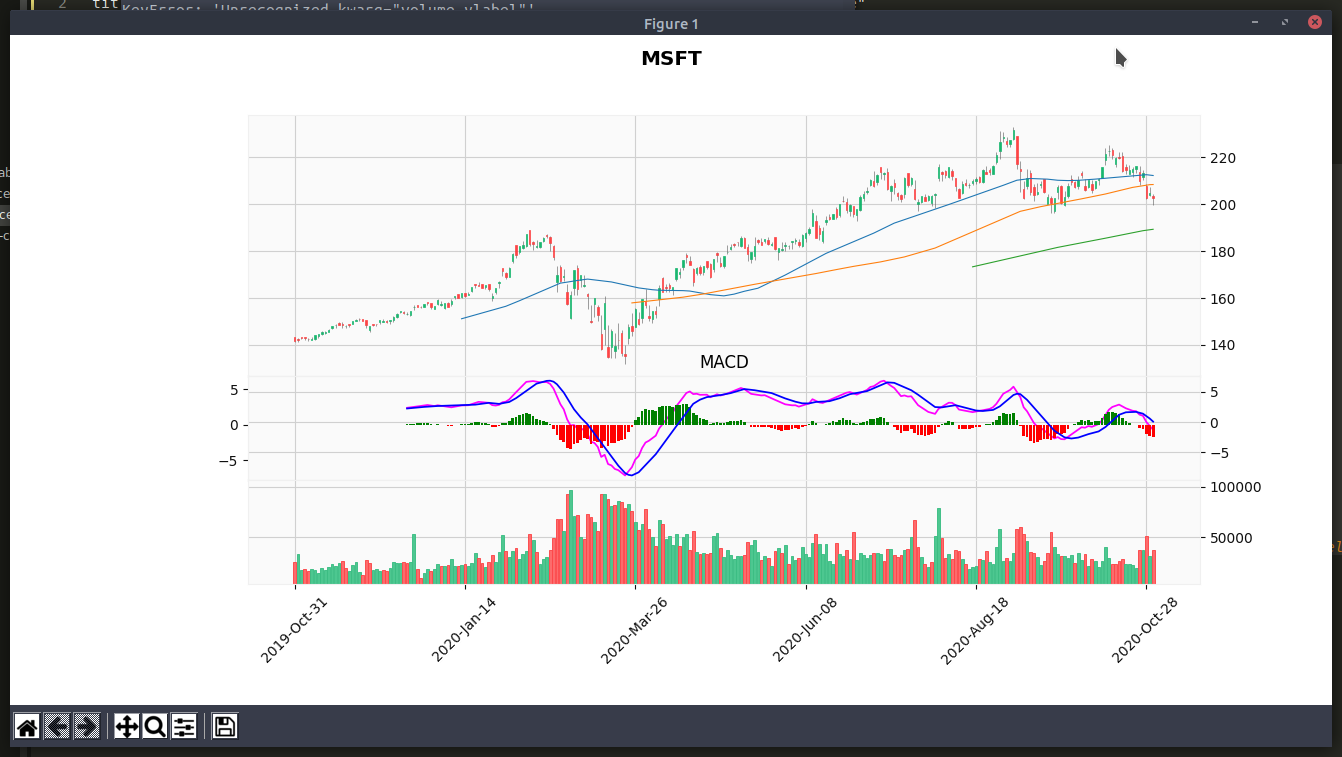# Python mplfinance Plot yfinance Candle Chart, Moving Average, MACD and Volume (Multi Panel)

Nov 2, 2020
``import yfinance as yfimport mplfinance as mpfimport talib as taticker_name = 'MSFT'yticker = yf.Ticker("MSFT")data = yticker.history(period="1y") # max, 1y, 3mo# trim volume to avoid exponential formdata['Volume'] = data['Volume'] / 1000# macddata["macd"], data["macd_signal"], data["macd_hist"] = ta.MACD(data['Close'])# macd panelcolors = ['g' if v >= 0 else 'r' for v in data["macd_hist"]]macd_plot = mpf.make_addplot(data["macd"], panel=1, color='fuchsia', title="MACD")macd_hist_plot = mpf.make_addplot(data["macd_hist"], type='bar', panel=1, color=colors) # color='dimgray'macd_signal_plot = mpf.make_addplot(data["macd_signal"], panel=1, color='b')# plotplots = [macd_plot, macd_signal_plot, macd_hist_plot]mpf.plot(data, type='candle', style='yahoo', mav=(50,100,200), addplot=plots, title=f"\n{ticker_name}", volume=True, volume_panel=2, ylabel='', ylabel_lower='')``